﻿ 基于CFD-DEM耦合数值模拟的全尺寸直升机沙盲形成机理Download PDF文章快速检索 高级检索

1. 南京航空航天大学 直升机旋翼动力学国家级重点实验室, 南京 210016;
2. 中国直升机设计研究所, 景德镇 333000

Formation mechanism of brownout in full-scale helicopter based on CFD-DEM couplings numerical simulation
HU Jianping1, XU Guohua1, SHI Yongjie1, WU Linbo2
1. National Key Laboratory of Science and Technology on Rotorcraft Aerodynamics, Nanjing University of Aeronautics and Astronautics, Nanjing 210016, China;
2. China Helicopter Research and Development Institute, Jingdezhen 333000, China
Abstract: To study the state and distribution of dust cloud in the hovering flow field of helicopter brownout, a numerical model based on Reynolds-averaged Navier-Stokes equations and Menter Shear Stress Transport (SST) k-ω turbulence model and a discrete element model based on Hertz-Mindlin (No Slip) contact model are coupled through the application programming interface. A more realistic model is constructed based on the multi-sphere method, and non-spherical dust particles are used to calculate the motion and distribution in the flow field. The effectiveness of the approach is verified by comparing the numerical results with the available experimental results. The drag coefficient of the rotor in the in-ground effect, the position of the tip vortices and the macroscopic outline of the dust cloud are calculated. By using the established method, the in-ground effect flow field of helicopters at different hovering altitudes is calculated. The vorticity and velocity contour of the flow field are obtained. The velocity and distribution of dust particles in dust clouds at different hovering altitudes are compared. The influence of ground effect flow field on the state of dust particles and the formation mechanism of dust clouds are analyzed. The macroscopic distribution of dust clouds are also obtained. The results show that most of the dust particles in the flow field can only diffuse with the flow field on the ground, but can not form dust clouds. The concentration of dust in outer space is higher than that in inner space. The dust particles located in the lower region of the rotor disk move mainly in the radial direction with smaller tangential velocity, while the velocity of the dust particles located in the upper region of the rotor disk are different in direction and close in magnitude.
Keywords: ground effect    brownout    CFD    helicopter    coupling discrete element method

“沙盲(Brownout)”是指当直升机在干燥，含有大量沙尘颗粒、雪花等离散相的近地面飞行时，沙尘被卷起形成沙尘云，严重阻碍飞行员视线、进而诱发严重事故的特殊现象[1-4](图 1)。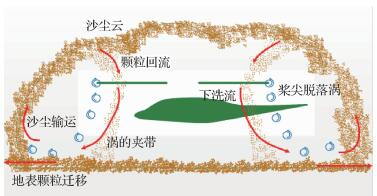图 1 直升机悬停时沙尘云中沙尘颗粒的宏观分布 Fig. 1 Macroscopic distribution of dust particlesin dust cloud during helicopter hover

1 CFD-DEM数值计算方法 1.1 CFD求解器和湍流模型

 $\mathit{\pmb{{\Gamma}}} \frac{\partial}{\partial t} \int\limits_\boldsymbol{V} \boldsymbol{Q} \mathrm{d} \boldsymbol{V}+\oint(\boldsymbol{F}-\boldsymbol{G}) \cdot \mathrm{d} \boldsymbol{a}=\int\limits_{\boldsymbol{V}} \boldsymbol{H} \mathrm{d} \boldsymbol{V}$ （1）

 $\boldsymbol{V}_{0} \mathit{\pmb{{\Gamma}}}_{0} \frac{\partial \boldsymbol{Q}_{0}}{\partial t}+\sum\limits_{f}\left(\boldsymbol{f}_{f}-\boldsymbol{g}_{f}\right) \cdot \boldsymbol{a}=\boldsymbol{h} \boldsymbol{V}_{0}$ （2）

1.2 网格系统和物理模型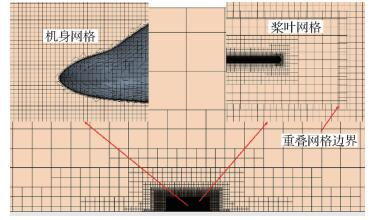图 2 重叠网格系统 Fig. 2 Overset grid system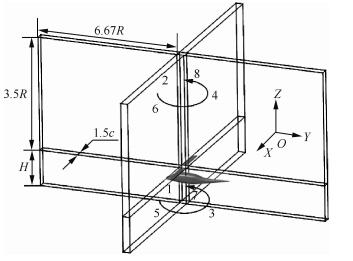图 3 区域划分 Fig. 3 Regional division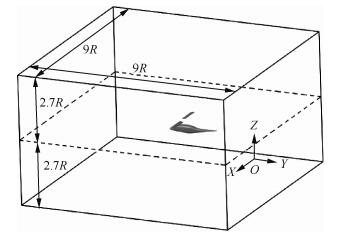图 4 上、下层区域划分 Fig. 4 Division of upper and lower regions
1.3 DEM求解器和沙尘颗粒物理模型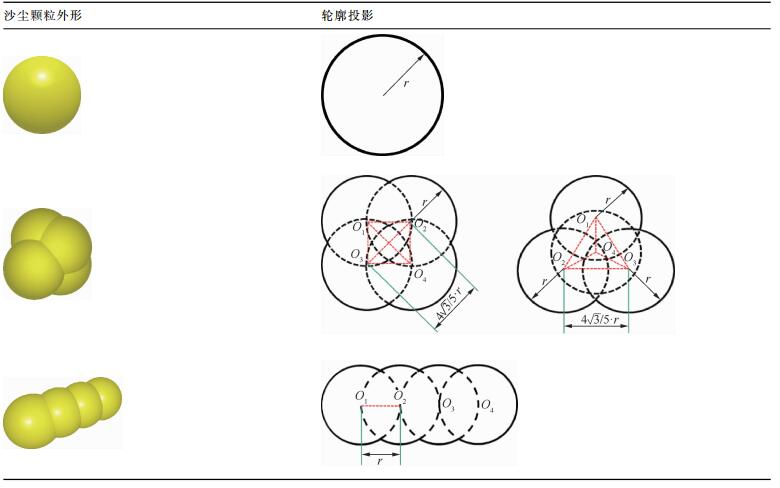材料 颗粒密度/(kg·m-3) 泊松比 剪切模量/Pa 恢复系数 静摩擦系数 滚动摩擦系数 沙尘颗粒 1 400 0.5 1×108 0.5 0.4 0.05 桨叶，机身 2 700 0.3 7×1010
1.4 CFD-DEM耦合求解模型

 $F_{\text {contact }}=F_{\mathrm{n}}+F_{\mathrm{t}}$ （3）

 $F_{\mathrm{n}}=-K_{\mathrm{n}} d_{\mathrm{n}}-N_{\mathrm{n}} v_{\mathrm{n}}$ （4）
 $F_{\mathrm{t}}=\left\{\begin{array}{ll} -K_{\mathrm{t}} d_{\mathrm{t}}-N_{\mathrm{t}} v_{\mathrm{t}} & \text { if }\left|K_{\mathrm{t}} d_{\mathrm{t}}\right|<\left|K_{\mathrm{n}} d_{\mathrm{n}}\right| C_{f{\mathrm{s}}} \\ \frac{\left|K_{\mathrm{t}} d_{\mathrm{t}}\right| C_{f_{\mathrm{s}}} d_{\mathrm{t}}}{\left|d_{\mathrm{t}}\right|} \end{array}\right.$ （5）

 $F_{\mathrm{JKR}}=-4 \sqrt{\pi \gamma E^{*}} \alpha^{3 / 2}+\frac{4 E^{*}}{3 R^{*}} \alpha^{3}$ （6）
 $\delta=\frac{\alpha^{2}}{R^{*}}-\sqrt{4 \pi \gamma \alpha / E^{*}}$ （7）

 $F_{\mathrm{drag}}=0.5 C_{\mathrm{d}} \rho_{\mathrm{g}} A\left|v_{\mathrm{p}-\mathrm{g}}^{\mathrm{rel}}\right| v_{\mathrm{p}-\mathrm{g}}^{\mathrm{rel}}$ （8）
 $C_\text{d}=\\ \left\{\begin{array}{ll} 24 / R e & R e \leqslant 0.5 \\ 24\left(1.0+0.15 R e^{0.687}\right) / R e & 0.51000 \end{array}\right.$ （9）
 $R e=\rho v L / \mu$ （10）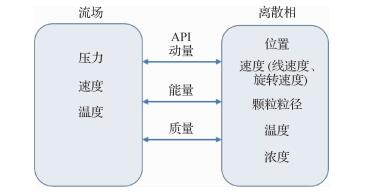图 5 流场和离散相之间通过API传递数据的过程 Fig. 5 Process of data transferring between flowfield and discrete phase through API
2 计算方法验证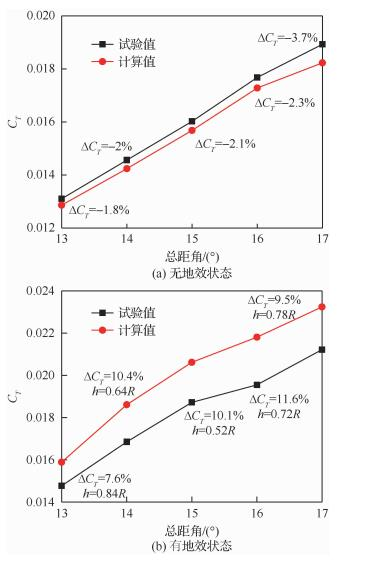图 6 拉力系数的试验值和计算值对比 Fig. 6 Comparison of experimental and numericalthrust coefficients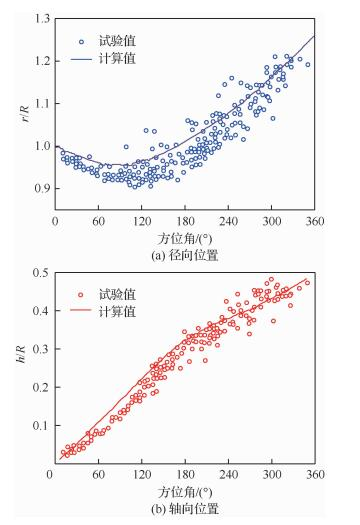图 7 桨尖涡位置的试验值和计算值对比 Fig. 7 Comparison of experimental and numericalblade tip vortex location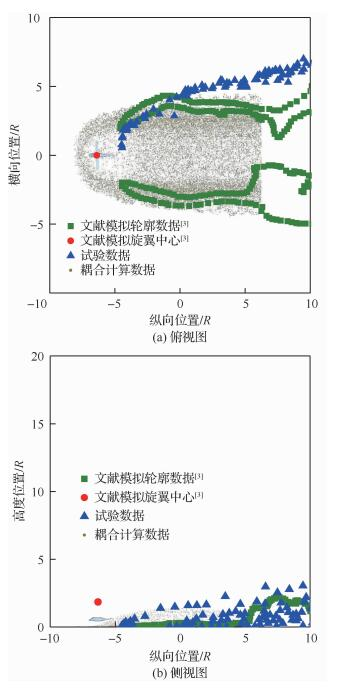图 8 沙尘云的试验测量和耦合数值模拟对比(t=10 s) Fig. 8 Comparison of dust cloud for experimentalmeasurements and coupling numericalsimulation (t=10 s)
3 沙尘云中沙尘颗粒运动规律分析 3.1 直升机地效流场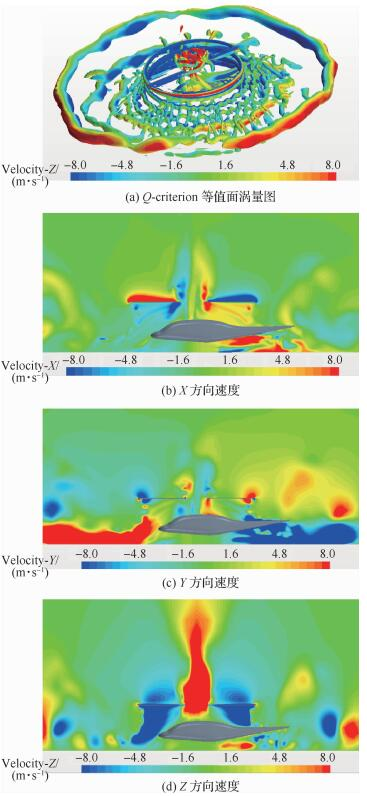图 9 有地效流场中涡量图和X、Y、Z方向速度云图 Fig. 9 Vorticity structure colored by Z-velocity andX, Y, Z directional velocity contours withIGE flow field
3.2 沙尘云中沙尘颗粒的运动规律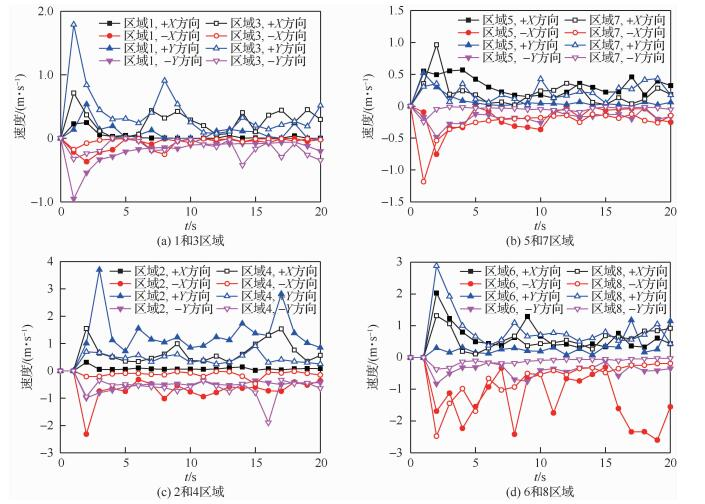图 10 H=0.67R时不同区域中沙尘颗粒在X和Y方向的平均速度 Fig. 10 Average velocities in X and Y directions of dust particles in different regions at H=0.67R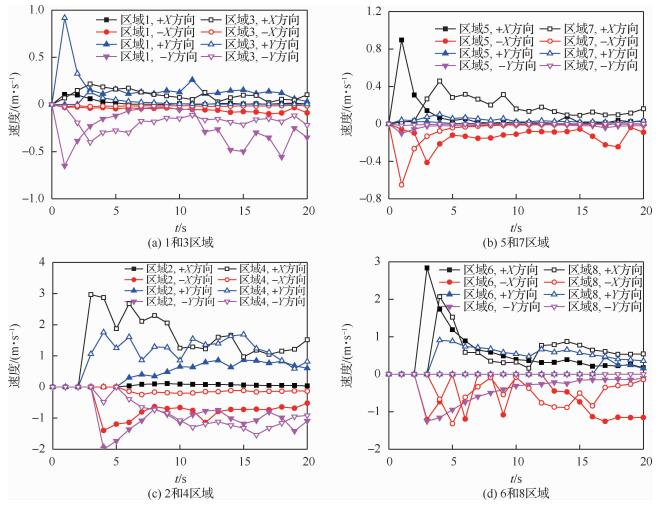图 11 H=1R时不同区域中沙尘颗粒在X和Y方向的平均速度 Fig. 11 Average velocities in X and Y directions of dust particles in different regions at H=1R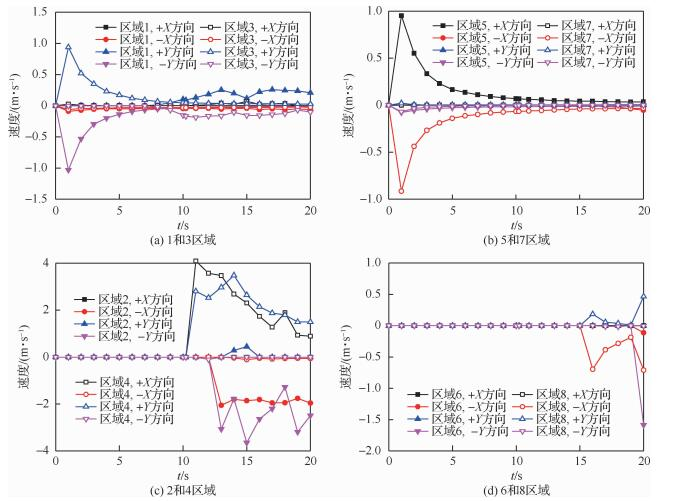图 12 H=1.5R时不同区域中沙尘颗粒在X和Y方向的平均速度 Fig. 12 Average velocities in X and Y directions of dust particles in different regions at H=1.5R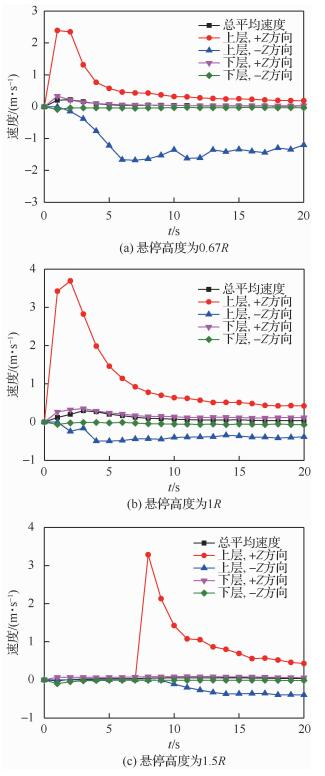图 13 不同悬停高度时沙尘颗粒在Z方向上的平均速度 Fig. 13 Average velocity of dust particles in Z direction at different hovering heights
3.3 沙尘云中沙尘颗粒的分布规律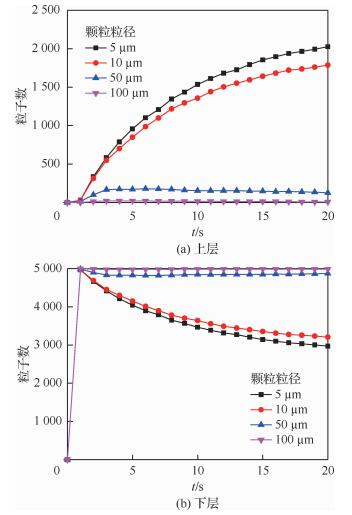图 14 H=0.67R时不同粒径沙尘颗粒数量分布 Fig. 14 Quantity distribution of dust particles withdifferent sizes at H=0.67R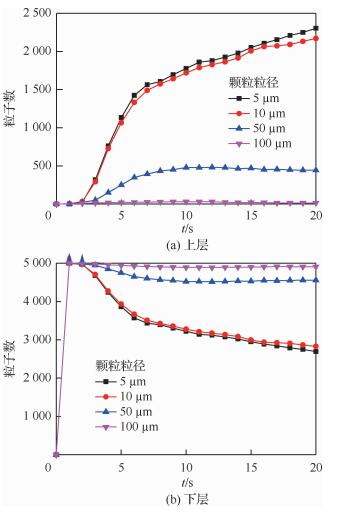图 15 H=1R时不同粒径沙尘颗粒数量分布 Fig. 15 Quantity distribution of dust particles withdifferent sizes at H=1R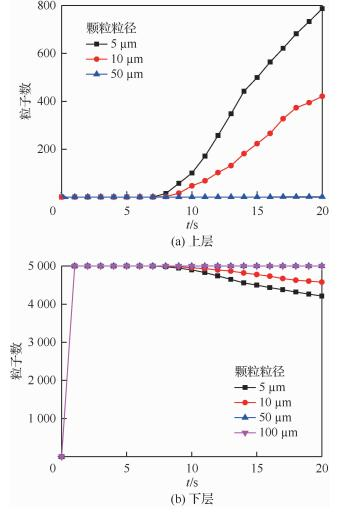图 16 H=1.5R时不同粒径沙尘颗粒数量分布 Fig. 16 Quantity distribution of dust particles withdifferent sizes at H=1.5R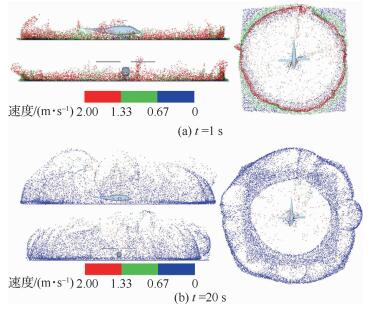图 17 H=0.67R时沙尘颗粒的宏观分布图 Fig. 17 Macroscopic distribution of dust particles at H=0.67R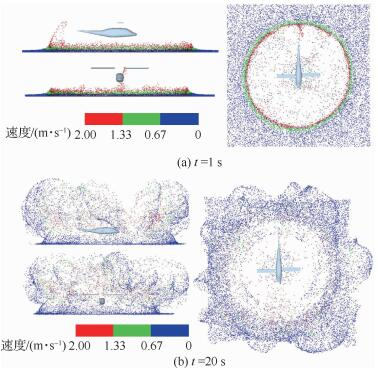图 18 H=1R时沙尘颗粒的宏观分布图 Fig. 18 Macroscopic distribution of dust particles at H=1R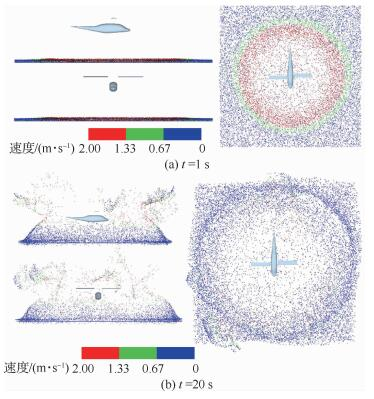图 19 H=1.5R时沙尘颗粒的宏观分布图 Fig. 19 Macroscopic distribution of dust particles atH=1.5R

4 结论

1) 沙尘云中大部分沙尘颗粒并未受流场的影响而被提升至上层区域，只在地表附近扩散。沙尘云径向范围随着悬停高度的升高而逐渐减小，但轴向范围变化不大。

2) 沙盲现象发生时，沙尘云中下层的沙尘颗粒主要做径向运动，而切向运动较弱，这种趋势随着悬停高度的增加变得更加显著。上层区域沙尘颗粒在桨盘平面XY方向上的平均速度波动较大，且无明显规律可循。

3) 沙尘云中初始到达上层区域的沙尘颗粒在轴向只有+Z方向的速度，随后受到下洗流的影响出现-Z方向的速度，随着时间的发展，+Z方向速度急剧减小，同时-Z方向速度先增加而后逐渐稳定。

4) 沙尘云形成初期阶段，地面处的沙尘颗粒由内向外扩散，随着时间的发展，沙尘云内层的沙尘颗粒仍然具有较大速度，而外层的沙尘颗粒速度逐渐减小，并随着沙尘云范围逐渐扩大而趋于悬浮在上层区域。

5) 位于沙尘云内层空间(旋翼及机身周围)的沙尘浓度较低，位于沙尘云外层空间的沙尘浓度较高。

  POLZIN J W, GUNTUPALLI K, RAJAGOPALAN R G. Discrete blade model for rotorcraft brownout[C]//29th AIAA Applied Aerodynamics Conference. Reston, VA: AIAA, 2011.  SYAL M, LEISHMAN J G. Comparisons of predicted brownout dust clouds with photogrammetry measurements[C]//67th Annual Forum of the American Helicopter Society, 2011.  SYAL M, LEISHMAN J G. Modeling of bombardment ejections in the rotorcraft brownout problem[J]. AIAA Journal, 2013, 51(4): 849-866. Click to display the text  WONG O D, TANNER P E. Photogrammetric measurements of an EH-60L brownout cloud[C]//American Helicopter Society 66th Annual Forum Proceedings, 2010.  叶靓, 招启军, 徐国华. 基于非结构嵌套网格方法的旋翼地面效应数值模拟[J]. 航空学报, 2009, 30(5): 780-786. YE L, ZHAO Q J, XU G H. Numerical simulation on flowfield of rotor in ground effect based on unstructured embedded grid method[J]. Acta Aeronautica et Astronautica Sinica, 2009, 30(5): 780-786. (in Chinese) Cited By in Cnki (13) | Click to display the text  朱明勇, 招启军, 王博. 基于CFD和混合配平算法的直升机旋翼地面效应模拟[J]. 航空学报, 2016, 37(8): 2539-2551. ZHU M Y, ZHAO Q J, WANG B. Simulation of helicopter rotor in ground effect based on CFD method and hybrid trim algorithm[J]. Acta Aeronautica et Astronautica Sinica, 2016, 37(8): 2539-2551. (in Chinese) Cited By in Cnki (7) | Click to display the text  GANESH B, KOMERATH N. Study of ground vortex structure of rotorcraft in ground effect at low advance ratios[C]//24th AIAA Applied Aerodynamics Conference. Reston, VA: AIAA, 2006.  LEE T E, LEISHMAN J G, RAMASAMY M. Fluid dynamics of interacting blade tip vortices with a ground plane[J]. Journal of the American Helicopter Society, 2010, 55(2): 022005. Click to display the text  MILLUZZO J I. Effects of blade tip shape on rotor in-ground-effect aerodynamics[D]. Maryland: University of Maryland, 2012: 39-83.  HANCE B T. Effects of body shapes on rotor in-ground-effect aerodynamics[D]. Maryland: University of Maryland, 2012: 42-66.  WHITEHOUSE G R, WACHSPRESS D A, QUACKENBUSH T R, et al. Exploring aerodynamic methods for mitigating brownout[C]//American Helicopter Society 65th Annual Forum, 2009.  WHITEHOUSE G R, WACHSPRESS D A, QUACKENBUSH T R. Aerodynamic design of helicopter rotors for reduced brownout[C]//International Powered Lift Conference, 2010.  THOMAS S, AMIRAUX M, BAEDER J D. Modeling the two-phase flowfield beneath a hovering rotor on graphics processing units using a FVM-RANS hybrid methodology[C]//21st AIAA Computational Fluid Dynamics Conference. Reston, VA: AIAA, 2013.  YU H M, CHENG W M, WU L R, et al. Mechanisms of dust diffuse pollution under forced-exhaust ventilation in fully-mechanized excavation faces by CFD-DEM[J]. Powder Technology, 2017, 317: 31-47. Click to display the text  HOU X Y, DING T X, DENG Z Q, et al. Study of the creeping of irregularly shaped Martian dust particles based on DEM-CFD[J]. Powder Technology, 2018, 328: 184-198. Click to display the text  魏可可, 高霄鹏. 基于STAR-CCM+对5415船模的阻力预报[J]. 兵器装备工程学报, 2016, 37(9): 157-161. WEI K K, GAO X P. Resistance prediction of 5415 ship model based on STAR-CCM+[J]. Journal of Ordnance Equipment Engineering, 2016, 37(9): 157-161. (in Chinese) Cited By in Cnki (5) | Click to display the text  DUBUC L, CANTARITI F, WOODGATE M, et al. Solution of the unsteady Euler equations using an implicit dual-time method[J]. AIAA Journal, 1998, 36(8): 1417-1424. Click to display the text  QU Q L, WANG W, LIU P Q, et al. Airfoil aerodynamics in ground effect for wide range of angles of attack[J]. AIAA Journal, 2015, 53(4): 1048-1061. Click to display the text  QIN Y P, LIU P Q, QU Q L, et al. Numerical study of aerodynamic forces and flow physics of a delta wing in dynamic ground effect[J]. Aerospace Science & Technology, 2016, 51: 152-221. Click to display the text  QIN Y P, LIU P Q, QU Q L, et al. Wing/canard interference of a close-coupled canard configuration in static ground effect[J]. Aerospace Science & Technology, 2017, 69: 60-75. Click to display the text  KUMAR M, MURTHY V. Analysis of flow around multibladed rotor using CFD in the frequency region[C]//25th AIAA Applied Aerodynamics Conference. Reston, VA: AIAA, 2007.  NOROUZI H R, ZARGHAMI R, SOTUDEH-GHAREBAGH R, et al. Coupled CFD-DEM modeling (formulation, implementation and application to multiphase flows)CFD-DEM applications to multiphase flow[M]. .  杜俊.基于CFD-DEM方法的希相气力输送数值模拟研究[D].武汉: 武汉大学, 2015: 20-21. DU J. Simulation of dilute pneumatic conveying by CFD-DEM[D]. Wuhan: Wuhan University, 2015: 20-21 (in Chinese).  LIGHT J S. Tip vortex geometry of a hovering helicopter rotor in ground effect[J]. Journal of the American Helicopter Society, 1993, 38(2): 34-42. Click to display the text  KUTZ B M, KOWARSCH U, KEßLER M, et al. Numerical investigation of helicopter rotors in ground effect[C]//30th AIAA Applied Aerodynamics Conference. Reston, VA: AIAA, 2012.
http://dx.doi.org/10.7527/S1000-6893.2019.23363

0

#### 文章信息

HU Jianping, XU Guohua, SHI Yongjie, WU Linbo

Formation mechanism of brownout in full-scale helicopter based on CFD-DEM couplings numerical simulation

Acta Aeronautica et Astronautica Sinica, 2020, 41(3): 123363.
http://dx.doi.org/10.7527/S1000-6893.2019.23363